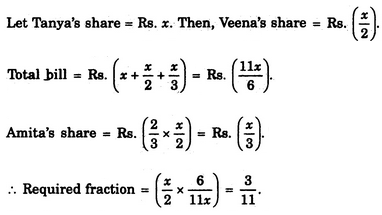# Verbal Reasoning - Arithmetic Reasoning - Discussion

### Discussion :: Arithmetic Reasoning - Section 1 (Q.No.36)

36.

Three friends had dinner at a restaurant. When the bill was received, Amita paid 2/3 as much as Veena paid and Veena paid 1/2 as much as Tanya paid. What faction of the bill did Veena pay ?

 [A]. 1/3 [B]. 3/11 [C]. 12/13 [D]. 5/8

Explanation:Brijesh said: (Dec 15, 2011) Let Tanya paid x Then Veena paid 1/2*x and Amita paid 2/3*1/2*x Tota bill (2/3*1/2*x)+ (1/2*x) + x = 1 2x+3x+6x = 6 x = 6/11 Veenna's Bill 1/2*6/11 = 3/11

 Rohit said: (Oct 22, 2014) Let Veena paid x, So Anita paid 2/3 x, And Tanya paid 2x, So total bill paid is given by, x+(2/3)x+2x = 1. i.e x = 3/11(ans).

 Kajal said: (Sep 21, 2017) Thanks @Rohit.

 Phanindra said: (Sep 6, 2018) @Rohit. How 2x came? Please explain.

 Shivam said: (Sep 20, 2018) As it is given that Veena paid 1/2 of what Tanya paid or simply Tanya paid double of Veena. That's why money paid by Tanya is assumed to be 2x.

 Navya Sree said: (May 10, 2019) Thanks @Rohit.

 Ram said: (May 14, 2022) Let, Tanay's share = x. Veena's share = x/2. Amita share = x/2*2/3=2x/6, Total amount = x+x/2+2x/6 = 1, =6x+3x+2x/6=1, =11x=6, X = 6/11. Veena share = x/2. = (6/11)/2, = 3/11.